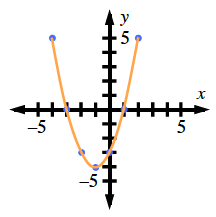### Home > CCG > Chapter 7 > Lesson 7.1.3 > Problem7-38

7-38.

Copy the table below on your paper and complete it for the equation $y=x^2+2x-3$. Then graph and connect the points on graph paper. Name the roots ($x$-intercepts).

 $x$ $y$ $-4$ $-3$ $-2$ $-1$ $0$ $1$ $2$

For $x=-4$,

$y=(-4)^2+2(-4)-3$

$y=5$

Now solve for $y$ in terms of the other $x$ values that have been given in the above table.

Make a graph by plotting the points from the table you have completed.Does yours look like the graph above?

Look for the two points of the parabola that touch the $x$-axis in order to find the $x$-intercepts.

Use the eTool below to complete the table and view the graph.
Click the link at right for the full version of the eTool: 7-38 HW eTool